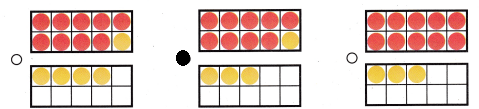Refer to our Texas Go Math Grade 1 Answer Key Pdf to score good marks in the exams. Test yourself by practicing the problems from Texas Go Math Grade 1 Module 7 Assessment Answer Key.

Vocabulary

Question 1.
Which fact do you use count back to solve? Circle it. (p.258)
8 – 2 = ___
1 + 7 = ___
8 – 2 = 6
1 + 7 = 8
Explanation:

Concepts and Skills

Use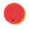to solve.

Draw to show your work. TEKS 1.3.D

Question 2.
What is 12 – 3?
12 – 3 = __Explanation:
Counted back to find the difference
The difference of 9 = 12 – 3

Count back 1, 2, or 3 to subtact.
Write the difference. TEKS 1.3.D

Question 3.
___ = 7 – 3Explanation:
Counted back to find the difference
The difference of 4 = 7 – 3

Question 4.
10 – 2 = ___Explanation:
Counted back to find the difference
The difference of 10 – 2 = 8

Question 5.
10 – 1 = ___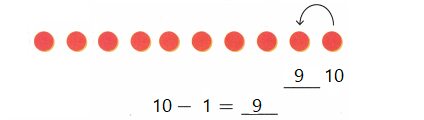Explanation:
Counted back to find the difference
The difference of 10 – 1 = 9

Question 6.
__ = 8 – 2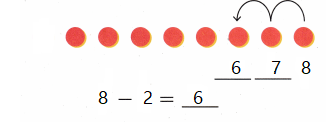Explanation:
Counted back to find the difference
The difference of 8 – 2 = 6

Question 7.
___ = 9 – 2Explanation:
Counted back to find the difference
The difference of 9 – 2 = 7

Question 8.
11 – 3 = ___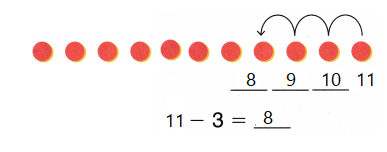Explanation:
Counted back to find the difference
The difference of 11 – 3 = 8

Question 9.
6 – 2 = ___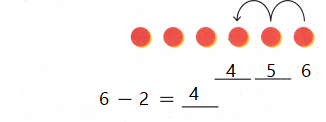Explanation:
Counted back to find the difference
The difference of 6 – 2 = 4

Question 10.
__ = 4 – 1Explanation:
Counted back to find the difference
The difference of 3 = 4 – 1

Texas Test Prep

Question 11.
What is 11 – 5? TEKS 1.3.D(A) 11
(B) 5
(C) 6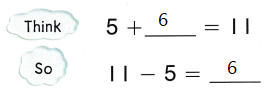Explanation:
11 – 5 = 6
5 + 6 = 11
Addition fact is used to find the subtraction fact

Question 12.
What subtraction sentence does the model show? TEKS 1.3.D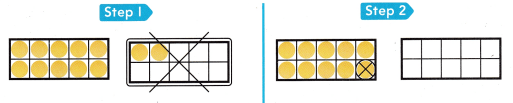(A) 12 – 3 = 9
(B) 10 – 1 = 9
(C) 12 – 2 = 10
Explanation:
12 – 3 = 9
To break a ten 3 are remaining
In step 1 two are crossed
and in step 2, 1 is crossed

Question 13.
Elsa had some marbles. She gave 9 marbles away. Now she has 9 marbles. How many marbles did Elsa start with? TEKS 1.3.D
(A) 9
(B) 0
(C) 18
Explanation:
18 – 9 = 9

Question 14.
Which shows a way to make a ten to subtract? TEKS 1.3.D
13 – 9 = ?